# A Multivariate Time Series Guide to Forecasting and Modeling (with Python codes)

## Introduction

Time is the most critical factor that decides whether a business will rise or fall. That’s why we see sales in stores and e-commerce platforms aligning with festivals. These businesses analyze years of spending data to understand the best time to throw open the gates and see an increase in consumer spending.

But how can you, as a data scientist, perform this analysis? Don’t worry, you don’t need to build a time machine! Time Series modeling is a powerful technique that acts as a gateway to understanding and forecasting trends and patterns.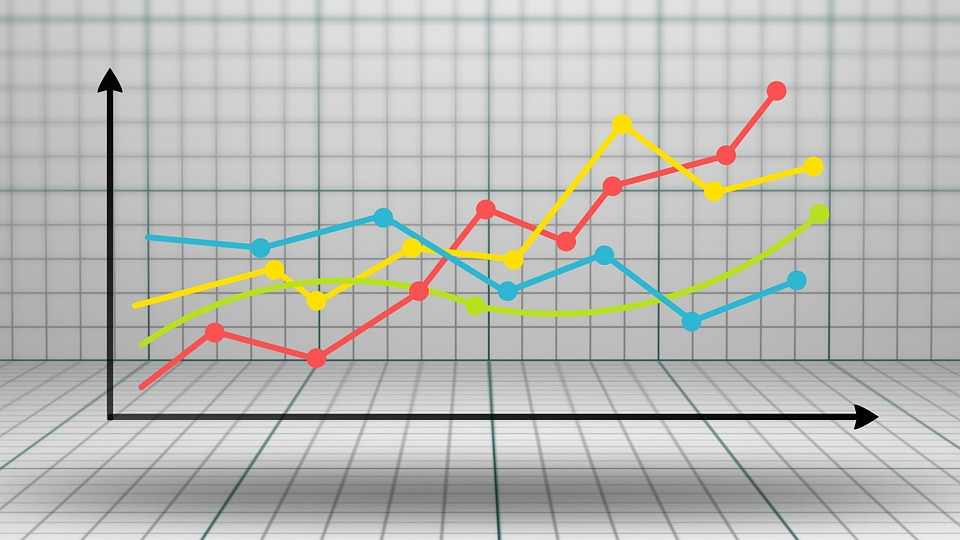But even a time series model has different facets. Most of the examples we see on the web deal with univariate time series. Unfortunately, real-world use cases don’t work like that. There are multiple variables at play, and handling all of them at the same time is where a data scientist will earn his worth.

In this article, we will understand what a multivariate time series is, and how to deal with it. We will also take a case study and implement it in Python to give you a practical understanding of the subject.

1. Univariate versus Multivariate Time Series
1. Univariate Time Series
2. Multivariate Time Series
2. Dealing with a Multivariate Time Series – Vector Auto Regression (VAR)
3. Why Do We Need VAR?
4. Stationarity in a Multivariate Time Series
5. Train-Validation Split
6. Python Implementation

## 1. Univariate versus Multivariate Time Series

This article assumes some familiarity with univariate time series, its properties and various techniques used for forecasting. Since this article will be focused on multivariate time series, I would suggest you go through the following articles which serve as a good introduction to univariate time series:

But I’ll give you a quick refresher of what a univariate time series is, before going into the details of a multivariate time series. Let’s look at them one by one to understand the difference.

### 1.1 Univariate Time Series

A univariate time series, as the name suggests, is a series with a single time-dependent variable.

For example, have a look at the sample dataset below that consists of the temperature values (each hour), for the past 2 years. Here, temperature is the dependent variable (dependent on Time).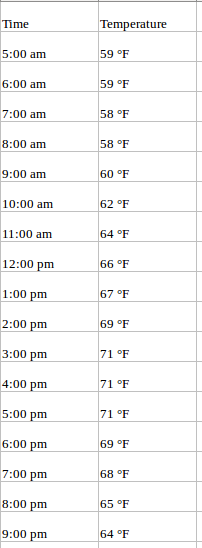If we are asked to predict the temperature for the next few days, we will look at the past values and try to gauge and extract a pattern. We would notice that the temperature is lower in the morning and at night, while peaking in the afternoon. Also if you have data for the past few years, you would observe that it is colder during the months of November to January, while being comparatively hotter in April to June.

Such observations will help us in predicting future values. Did you notice that we used only one variable (the temperature of the past 2 years,)? Therefore, this is called Univariate Time Series Analysis/Forecasting.

### 1.2 Multivariate Time Series (MTS)

A Multivariate time series has more than one time-dependent variable. Each variable depends not only on its past values but also has some dependency on other variables. This dependency is used for forecasting future values. Sounds complicated? Let me explain.

Consider the above example. Now suppose our dataset includes perspiration percent, dew point, wind speed, cloud cover percentage, etc. along with the temperature value for the past two years. In this case, there are multiple variables to be considered to optimally predict temperature. A series like this would fall under the category of multivariate time series. Below is an illustration of this: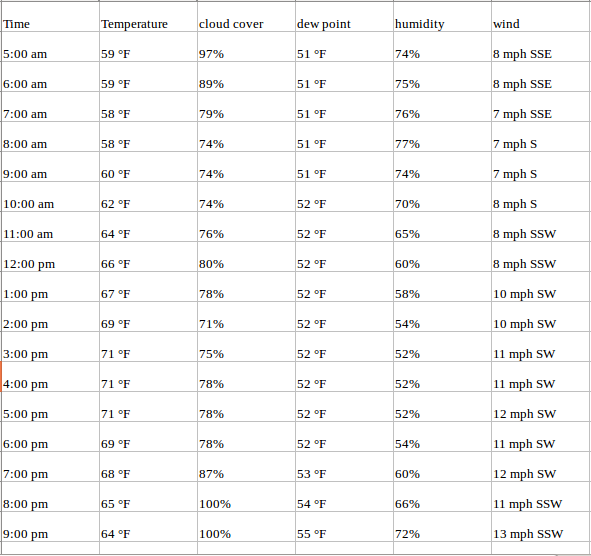Now that we understand what a multivariate time series looks like, let us understand how can we use it to build a forecast.

## 2. Dealing with a Multivariate Time Series – VAR

In this section, I will introduce you to one of the most commonly used methods for multivariate time series forecasting – Vector Auto Regression (VAR).

In a VAR model, each variable is a linear function of the past values of itself and the past values of all the other variables. To explain this in a better manner, I’m going to use a simple visual example:

We have two variables, y1 and y2. We need to forecast the value of these two variables at time t, from the given data for past n values. For simplicity, I have considered the lag value to be 1.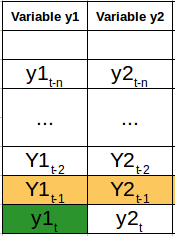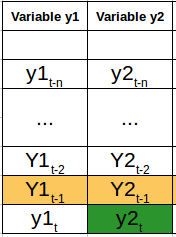For calculating y1(t), we will use the past value of y1 and y2. Similarly, to calculate y2(t), past values of both y1 and y2 will be used. Below is a simple mathematical way of representing this relation: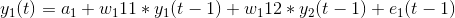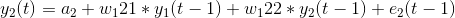Here,

• a1 and a2 are the constant terms,
• w11, w12, w21, and w22 are the coefficients,
• e1 and e2 are the error terms

These equations are similar to the equation of an AR process. Since the AR process is used for univariate time series data, the future values are linear combinations of their own past values only. Consider the AR(1) process:

y(t) = a + w*y(t-1) +e

In this case, we have only one variable – y, a constant term – a, an error term – e, and a coefficient – w. In order to accommodate the multiple variable terms in each equation for VAR, we will use vectors.  We can write the equations (1) and (2) in the following form :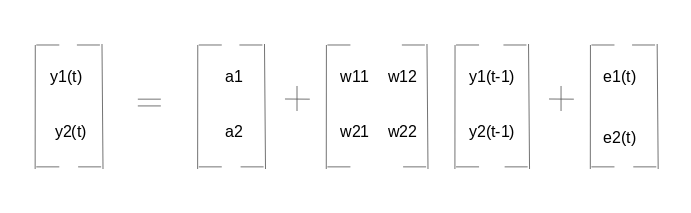The two variables are y1 and y2, followed by a constant, a coefficient metric, lag value, and an error metric. This is the vector equation for a VAR(1) process. For a VAR(2) process, another vector term for time (t-2) will be added to the equation to generalize for p lags: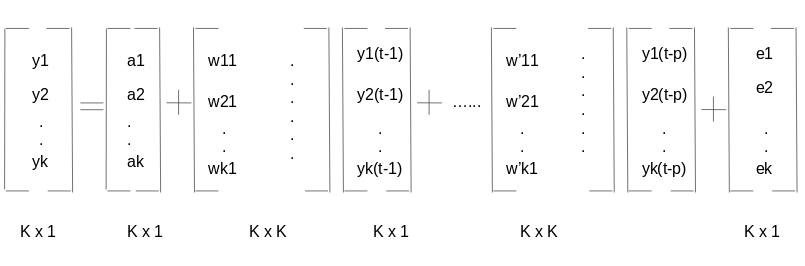The above equation represents a VAR(p) process with variables y1, y2 …yk. The same can be written as: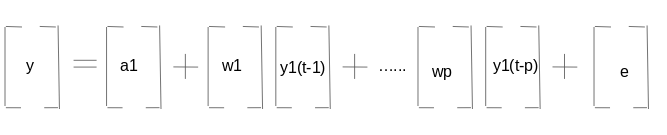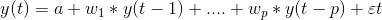The term εt in the equation represents multivariate vector white noise. For a multivariate time series, εshould be a continuous random vector that satisfies the following conditions:

1. E(εt) = 0
Expected value for the error vector is 0
2. E(εt1t2‘) = σ12
Expected value of εand εt‘ is the standard deviation of the series

## 3. Why Do We Need VAR?

Recall the temperate forecasting example we saw earlier. An argument can be made for it to be treated as a multiple univariate series. We can solve it using simple univariate forecasting methods like AR. Since the aim is to predict the temperature, we can simply remove the other variables (except temperature) and fit a model on the remaining univariate series.

Another simple idea is to forecast values for each series individually using the techniques we already know. This would make the work extremely straightforward! Then why should you learn another forecasting technique? Isn’t this topic complicated enough already?

From the above equations (1) and (2), it is clear that each variable is using the past values of every variable to make the predictions. Unlike AR, VAR is able to understand and use the relationship between several variables. This is useful for describing the dynamic behavior of the data and also provides better forecasting results. Additionally, implementing VAR is as simple as using any other univariate technique (which you will see in the last section).

## 4. Stationarity of a Multivariate Time Series

We know from studying the univariate concept that a stationary time series will more often than not give us a better set of predictions. If you are not familiar with the concept of stationarity, please go through this article first: A Gentle Introduction to handling non-stationary Time Series.

To summarize, for a given univariate time series:

y(t) = c*y(t-1) + ε t

The series is said to be stationary if the value of |c| < 1. Now, recall the equation of our VAR process: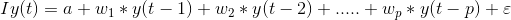Note: I is the identity matrix.

Representing the equation in terms of Lag operators, we have: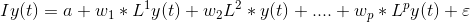Taking all the y(t) terms on the left-hand side: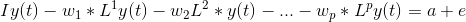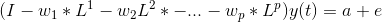The coefficient of y(t) is called the lag polynomial. Let us represent this as Φ(L):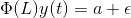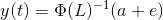For a series to be stationary, the eigenvalues of |Φ(L)-1| should be less than 1 in modulus. This might seem complicated given the number of variables in the derivation. This idea has been explained using a simple numerical example in the following video. I highly encourage watching it to solidify your understanding:

Similar to the Augmented Dickey-Fuller test for univariate series, we have Johansen’s test for checking the stationarity of any multivariate time series data. We will see how to perform the test in the last section of this article.

## 5. Train-Validation Split

If you have worked with univariate time series data before, you’ll be aware of the train-validation sets. The idea of creating a validation set is to analyze the performance of the model before using it for making predictions.

Creating a validation set for time series problems is tricky because we have to take into account the time component. One cannot directly use the train_test_split or k-fold validation since this will disrupt the pattern in the series. The validation set should be created considering the date and time values.

Suppose we have to forecast the temperate, dew point, cloud percent, etc. for the next two months using data from the last two years. One possible method is to keep the data for the last two months aside and train the model on the remaining 22 months.

Once the model has been trained, we can use it to make predictions on the validation set. Based on these predictions and the actual values, we can check how well the model performed, and the variables for which the model did not do so well. And for making the final prediction, use the complete dataset (combine the train and validation sets).

## 6. Python implementation

In this section, we will implement the Vector AR model on a toy dataset. I have used the Air Quality dataset for this and you can download it from here.

```#import required packages
import pandas as pd
import matplotlib.pyplot as plt
%matplotlib inline

df = pd.read_csv("AirQualityUCI.csv", parse_dates=[['Date', 'Time']])

#check the dtypes
df.dtypes```

```Date_Time        object
CO(GT)            int64
PT08.S1(CO)       int64
NMHC(GT)          int64
C6H6(GT)          int64
PT08.S2(NMHC)     int64
NOx(GT)           int64
PT08.S3(NOx)      int64
NO2(GT)           int64
PT08.S4(NO2)      int64
PT08.S5(O3)       int64
T                 int64
RH                int64
AH                int64
dtype: object```

The data type of the Date_Time column is object and we need to change it to datetime. Also, for preparing the data, we need the index to have datetime. Follow the below commands:

```df['Date_Time'] = pd.to_datetime(df.Date_Time , format = '%d/%m/%Y %H.%M.%S')
data = df.drop(['Date_Time'], axis=1)
data.index = df.Date_Time```

The next step is to deal with the missing values. Since the missing values in the data are replaced with a value -200, we will have to impute the missing value with a better number. Consider this – if the present dew point value is missing, we can safely assume that it will be close to the value of the previous hour. Makes sense, right? Here, I will impute -200 with the previous value.

You can choose to substitute the value using the average of a few previous values, or the value at the same time on the previous day (you can share your idea(s) of imputing missing values in the comments section below).

```#missing value treatment
cols = data.columns
for j in cols:
for i in range(0,len(data)):
if data[j][i] == -200:
data[j][i] = data[j][i-1]

#checking stationarity
from statsmodels.tsa.vector_ar.vecm import coint_johansen
#since the test works for only 12 variables, I have randomly dropped
#in the next iteration, I would drop another and check the eigenvalues
johan_test_temp = data.drop([ 'CO(GT)'], axis=1)
coint_johansen(johan_test_temp,-1,1).eig```

Below is the result of the test:

```array([ 0.17806667,  0.1552133 ,  0.1274826 ,  0.12277888,  0.09554265,
0.08383711,  0.07246919,  0.06337852,  0.04051374,  0.02652395,
0.01467492,  0.00051835])```

We can now go ahead and create the validation set to fit the model, and test the performance of the model:

```#creating the train and validation set
train = data[:int(0.8*(len(data)))]
valid = data[int(0.8*(len(data))):]

#fit the model
from statsmodels.tsa.vector_ar.var_model import VAR

model = VAR(endog=train)
model_fit = model.fit()

# make prediction on validation
prediction = model_fit.forecast(model_fit.y, steps=len(valid))```

The predictions are in the form of an array, where each list represents the predictions of the row. We will transform this into a more presentable format.

```#converting predictions to dataframe
pred = pd.DataFrame(index=range(0,len(prediction)),columns=[cols])
for j in range(0,13):
for i in range(0, len(prediction)):
pred.iloc[i][j] = prediction[i][j]

#check rmse
for i in cols:
print('rmse value for', i, 'is : ', sqrt(mean_squared_error(pred[i], valid[i])))```

Output of the above code:

```rmse value for CO(GT) is :  1.4200393103392812
rmse value for PT08.S1(CO) is :  303.3909208229375
rmse value for NMHC(GT) is :  204.0662895081472
rmse value for C6H6(GT) is :  28.153391799471244
rmse value for PT08.S2(NMHC) is :  6.538063846286176
rmse value for NOx(GT) is :  265.04913993413805
rmse value for PT08.S3(NOx) is :  250.7673347152554
rmse value for NO2(GT) is :  238.92642219826683
rmse value for PT08.S4(NO2) is :  247.50612831072633
rmse value for PT08.S5(O3) is :  392.3129907890131
rmse value for T is :  383.1344361254454
rmse value for RH is :  506.5847387424092
rmse value for AH is :  8.139735443605728
```

After the testing on validation set, lets fit the model on the complete dataset

```#make final predictions
model = VAR(endog=data)
model_fit = model.fit()
yhat = model_fit.forecast(model_fit.y, steps=1)
print(yhat)```

## End Notes

Before I started this article, the idea of working with a multivariate time series seemed daunting in its scope. It is a complex topic, so take your time in understanding the details. The best way to learn is to practice, and so I hope the above Python implemenattion will be useful for you.

I enocurage you to use this approach on a dataset of your choice. This will further cement your understanding of this complex yet highly useful topic. If you have any suggestions or queries, share them in the comments section.

Este sitio usa Akismet para reducir el spam. Aprende cómo se procesan los datos de tus comentarios.